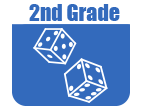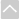OER Curriculum

These Open Educational Resources are comprehensive and coherent curricular materials that may be used to teach a course or grade level.

Engage NY
• Grade 2 Math Module 1: Sums and Differences to 100 (EngageNY)
Module 1 sets the foundation for students to master sums and differences to 20. Students subsequently apply these skills to fluently add one-digit to two-digit numbers at least through 100 using place value understanding, properties of operations, and the relationship between addition and subtraction.
• Grade 2 Math Module 2: Addition and Subtraction of Length Units (Engage NY)
In this 12-day Grade 2 module, students engage in activities designed to deepen their conceptual understanding of measurement and to relate addition and subtraction to length. Their work in Module 2 is exclusively with metric units in order to support place value concepts. Customary units will be introduced in Module 7.
• Grade 2 Math Module 3: Place Value, Counting, and Comparison of Numbers to 1,000 (EngageNY)
In this 25-day Grade 2 module, students expand their skill with and understanding of units by bundling ones, tens, and hundreds up to a thousand with straws. Unlike the length of 10 centimeters in Module 2, these bundles are discrete sets. One unit can be grabbed and counted just like a banana-1 hundred, 2 hundred, 3 hundred, etc. A number in Grade 1 generally consisted of two different units, tens and ones. Now, in Grade 2, a number generally consists of three units: hundreds, tens, and ones. The bundled units are organized by separating them largest to smallest, ordered from left to right. Over the course of the module, instruction moves from physical bundles that show the proportionality of the units to non-proportional place value disks and to numerals on the place value chart.
• Grade 2 Math Module 4: Addition and Subtraction Within 200 with Word Problems to 100 (EngageNY)
In Module 4, students develop place value strategies to fluently add and subtract within 100; they represent and solve one- and two-step word problems of varying types within 100; and they develop conceptual understanding of addition and subtraction of multi-digit numbers within 200. Using a concrete to pictorial to abstract approach, students use manipulatives and math drawings to develop an understanding of the composition and decomposition of units, and they relate these representations to the standard algorithm for addition and subtraction.
• Grade 2 Math Module 5: Addition and Subtraction Within 1,000 with Word Problems to 100 (Engage NY)
In Module 5, students build upon their mastery of renaming place value units and extend their work with conceptual understanding of the addition and subtraction algorithms to numbers within 1,000, always with the option of modeling with materials or drawings. Throughout the module, students continue to focus on strengthening and deepening conceptual understanding and fluency.
• Grade 2 Math Module 6: Foundations of Multiplication and Division (EngageNY)
Module 6 lays the conceptual foundation for multiplication and division in Grade 3 and for the idea that numbers other than 1, 10, and 100 can serve as units. Topics in this module include: Formation of Equal Groups, Arrays and Equal Groups, Rectangular Arrays as a Foundation for Multiplication and Division, and The Meaning of Even and Odd Numbers.
• Grade 2 Math Module 7: Problem Solving with Length, Money, and Data (Engage NY)
Module 7 presents an opportunity for students to practice addition and subtraction strategies within 100 and problem-solving skills as they learn to work with various types of units within the contexts of length, money, and data. Students represent categorical and measurement data using picture graphs, bar graphs, and line plots. They revisit measuring and estimating length from Module 2, though now using both metric and customary units.
• Grade 2 Math Module 8: Time, Shapes, and Fractions as Equal Parts of Shapes (Engage NY)
In Module 8, the final module of the year, students extend their understanding of partwhole relationships through the lens of geometry. As students compose and decompose shapes, they begin to develop an understanding of unit fractions as equal parts of a whole.
•Georgia Standards of Excellence Curriculum Frameworks
• Grade 2 Unit 1: Extending Base Ten Understanding (Georgia Standards)
In this unit, students will understand the value placed on the digits within a three-digit number, recognize that a hundred is created from ten groups of ten, use skip counting strategies to skip count by 5s, 10s, and 100s within 1,000 and represent numbers to 1,000 by using numbers, number names, and expanded form, compare two-digit number using >, =, <.
• Grade 2 Unit 2: Becoming Fluent with Addition and Subtraction (Georgia Standards)
In this unit students will cultivate an understanding of how addition and subtraction affect quantities and are related to each other, will reinforce the multiple meanings for addition (combine, join, and count on) and subtraction (take away, remove, count back, and compare), further develop their understanding of the relationships between addition and subtraction, recognize how the digits 0-9 are used in our place value system to create numbers and manipulate amounts and continue to develop their understanding solving problems with money.
• Grade 2 Unit 3: Understanding Measurement, Length, and Time (Georgia Standards)
In this unit students will know the following customary units for measuring length: inch, foot, yard, recognize the need for standard units of measure, understand the relationship of hours and days and nderstand the importance, usefulness of reasonable estimations and e able to represent the length of several objects by making a line plot.
• Grade 2 Unit 4: Applying Base Ten Understanding (Georgia Standards)
In this unit students will continue to develop their understanding of and facility with addition and subtraction, add up to 4 two-digit numbers, use a variety of models (base ten blocks- ones, tens, and hundreds only; diagrams; number lines; place value strategies; etc.) to add and subtract within one thousand and continue to develop their understanding of, and facility with, money.
• Grade 2 Unit 5: Understanding Plane and Solid Figures (Georgia Standards)
In this unit students will cultivate spatial awareness by further developing understandings of basic geometric figures, identifying plane figures and solid figures based on geometric properties, understand what an array is and how it can be used as a model for repeated addition and organize and record data using tallies, simple tables and charts, picture graphs, and bar graphs.
• Grade 2 Unit 6: Developing Multiplication (Georgia Standards)
In this unit students will understand and model multiplication as repeated addition and as rectangular arrays, determine if a number is odd or even (within twenty) and create and interpret picture graphs and bar graphs.
•The Online Core Resource pages are a collaborative project between the Utah State Board of Education and the Utah Education Network. If you would like to recommend a high quality resource, contact Trish French (Elementary) or Lindsey Henderson (Secondary). If you find inaccuracies or broken links contact resources@uen.org.